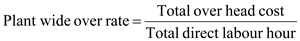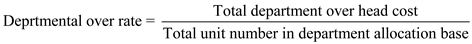# Quiz 7: Activity-Based Costing and Management

The two stage process associated with plant wide over rate is as follows: Stage1. In first stage, plant wide overhead rate depends on the direct labour hours. To find the overhead rate the total overhead cost is divided by total labour hours. In which we calculate the single plant wide overhead rate on cost allocation. After calculation, we get plant-wide overhead rate per direct labour.Stage2. In second stage, we calculate the total cost and also calculate per unit cost of each product.
The two stage process for departmental over rates is as follows: Stage1. In this stage, departmental overhead rate depends on the direct labour hours (indirect cost). In first situation, it calculates the departmental wise rate in which there are two departments. Stage2. In this stage, it calculates the overhead rate on the departmental wise rate, then it calculates the cost per unit of each product in which every product have relation with two departments.